# Selina Solutions Concise Mathematics Class 6 Chapter 25 Properties of Angles and Lines Exercise 25(D)

Selina Solutions Concise Mathematics Class 6 Chapter 25 Properties of Angles and Lines Exercise 25(D) has clear answers in an understandable language. The solutions prepared by experts help students in solving complex problems easily. Students clear their doubts about the concept on the spot, by referring to the solutions on a daily basis. The solved examples present in the chapter, help students to ace the examination. Those who aspire to become experts in solving complex problems within a short span are suggested to practice Selina Solutions repeatedly. For a better hold on the subject, students can refer to Selina Solutions Concise Mathematics Class 6 Chapter 25 Properties of Angles and Lines Exercise 25(D) PDF, from the links which are available below.

## Selina Solutions Concise Mathematics Class 6 Chapter 25: Properties of Angles and Lines Exercise 25(D) Download PDF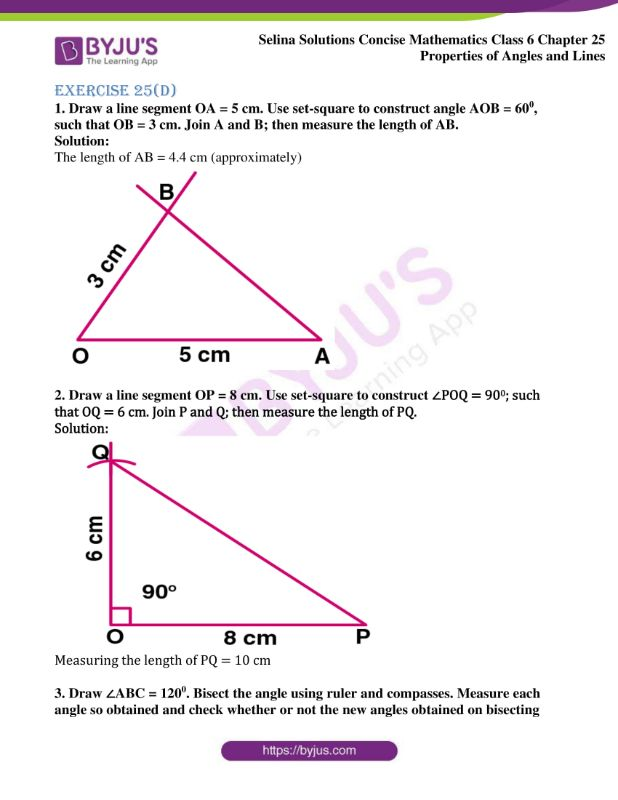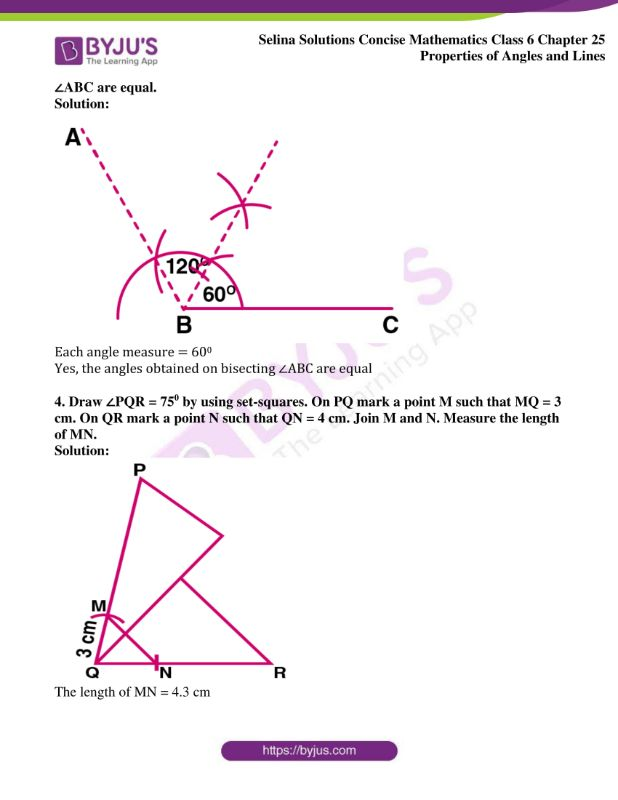### Access Selina Solutions Concise Mathematics Class 6 Chapter 25: Properties of Angles and Lines Exercise 25(D)

Exercise 25(D)

1. Draw a line segment OA = 5 cm. Use set-square to construct angle AOB = 600, such that OB = 3 cm. Join A and B; then measure the length of AB.

Solution:

The length of AB = 4.4 cm (approximately)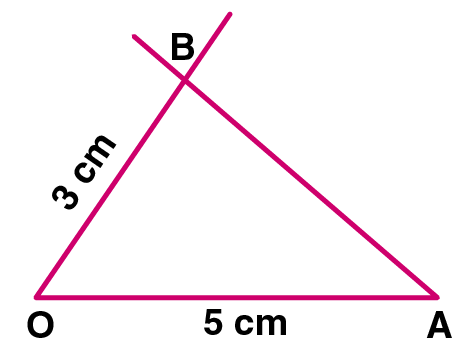2. Draw a line segment OP = 8 cm. Use set-square to construct ∠POQ = 900; such that OQ = 6 cm. Join P and Q; then measure the length of PQ.

Solution: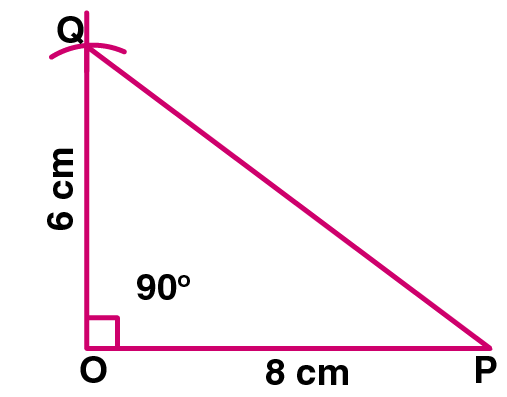Measuring the length of PQ = 10 cm

3. Draw ∠ABC = 1200. Bisect the angle using ruler and compasses. Measure each angle so obtained and check whether or not the new angles obtained on bisecting ∠ABC are equal.

Solution: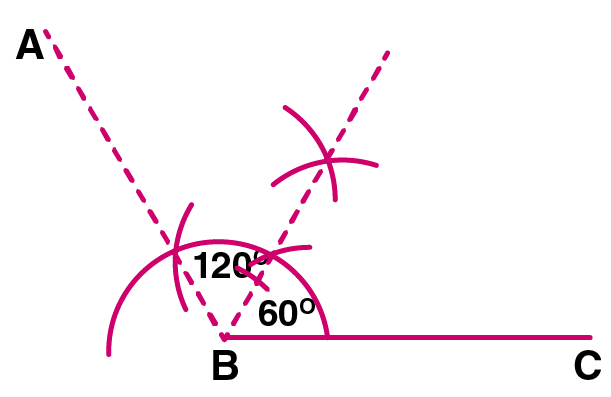Each angle measure = 600

Yes, the angles obtained on bisecting ∠ABC are equal

4. Draw ∠PQR = 750 by using set-squares. On PQ mark a point M such that MQ = 3 cm. On QR mark a point N such that QN = 4 cm. Join M and N. Measure the length of MN.

Solution: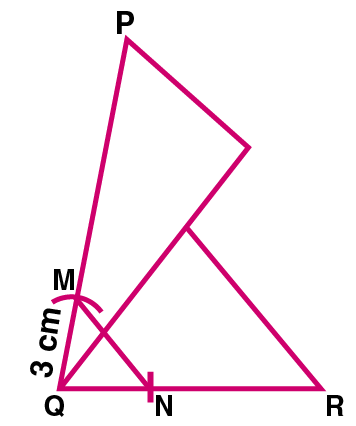The length of MN = 4.3 cm